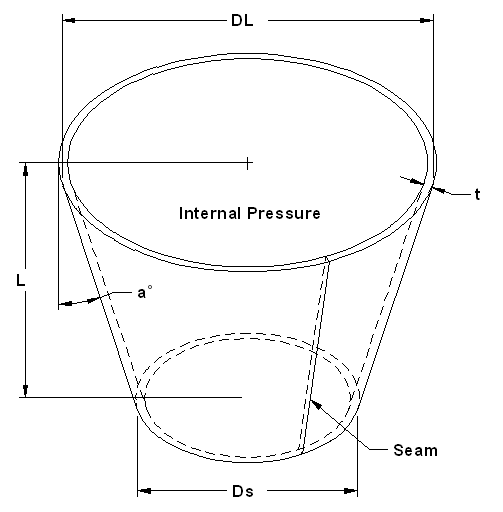Pressure Vessel Pipe Stress Cone Stress and Pressure Design Calculator

This calculator is based on VIII-1, UG-32 and is applied to a conical section.

UG-16(b) minimum thickness requirement has not been taken into consideration here.
 Wall Thickness (t)Pressure (P)Variables: OL [in] - large outside diameter Os [in] - small outside diameter t [in] - thickness L [in] -length Cr [in] - corrosion allowance factor S [psi] - allowable material stress E - seam efficiency (weld) P [psi] - internal pressure Calculated Data: a [rad] = a [deg] = nt [in] = Required Material Thickness: Tr [in] = Tr vs t Maximum Pressure: Pm [psi] = Pm vs P

Where:

E = seam (joint) efficiency for, or the efficiency of, appropriate joint in cylindrical or spherical shells, or the efficiency of ligaments between openings, whichever is less.
For welded vessels, use the efficiency specified in UW-12. For ligaments between openings, use the efficiency calculated by the rules given in UG-53.

P = internal design pressure (see UG-21)

OL = diameter of large end of cone

Os = diameter of small end of cone

S = maximum allowable stress value (see UG-23 and the stress limitations specified in UG-24)

t = design thickness of cone shell

Cr = corrosion allowancefactor

Tr = required thickness of cone shell

Pm = Pressure maximum (psi)

a = atan(OL - Os)/(2L) (radians) calculated angle

nt = t - cr (thickness of cone plate compensated for corrosions allowance)

References: ASME Boiler and Pressure Vessel Code

Contributed by:

Harsh Murugesan
Pune, Maharashtra IndiaMembership Register | LoginHomeEngineering Book StoreEngineering ForumExcel App. DownloadsOnline Books & ManualsEngineering NewsEngineering VideosEngineering CalculatorsEngineering ToolboxGD&T Training Geometric Dimensioning TolerancingDFM DFA TrainingTraining Online EngineeringAdvertising CenterCopyright Notice